# 7th Grade - Angles & Triangles

## Introduction

Angles are formed by rays that intersect at the same vertex.

A ray is part of a line with a fixed starting point extending infinitely in the other direction.

Angles are labeled by both rays (lines). The vertex is in the middle of the label. Rays $\stackrel{⇀}{AO}$ and $\stackrel{⇀}{OB}$ intersect at point O, which is the vertex, to form angle AOB.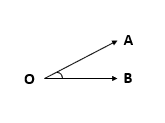## Types of Angles

There are six different types of angles.

1. Acute Angle

• measures less than 90o
• the measure of angle AOB shown below is less than 90o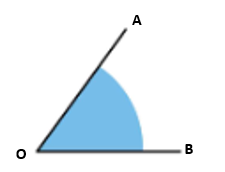2. Right Angle

• measures exactly 90o
• the measure of angle MOP shown below is 90o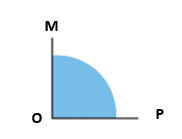3. Obtuse Angle

• measures greater than 90o but less than 180o
• the measure of angle RST shown below is greater than 90o but less than 180o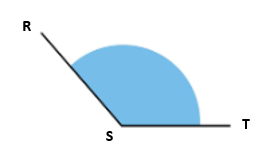4. Straight Angle

• measures exactly 180o
• the measure of angle PQR shown below is 180o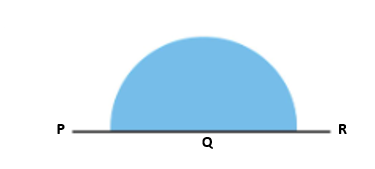5. Reflex Angle

• measures greater than 180o but less than 360o
• the measure of angle XYZ shown below is greater than 180o but less than 360o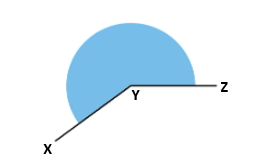6. Full Rotation

• measures exactly 360o
• the measure of angle ABC shown below is 360o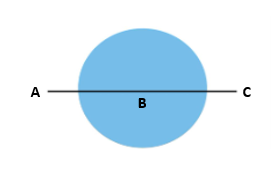## Basic Concepts of Polygons, Triangles, Interior Angles, and Exterior Angles

• A triangle is a two-dimensional plane figure or a polygon with three sides.
• Triangles can be classified based on angles and side lengths. In general, we only have two types of triangles - The Right Triangle and the Oblique Triangle.
• Polygons may be classified as follows:
1. A simple polygon is a type of polygon consisting of straight, non-intersecting line segments or sides that are connected to form a shape.
2. A complex polygon is a type of polygon that intersects itself.
3. A convex polygon is a polygon that is having any line segment connecting the interior of a figure is part of the region.
4. A concave (non-convex) polygon is a polygon that has an interior angle greater than $180°$.
5. A regular polygon is a polygon that is both equilateral (all sides are congruent) and equiangular (all interior angles are congruent).
6. An irregular polygon is a polygon wherein all angles are not congruent, and sides have different measures.
• We can compute the sum of all interior angles of a convex polygon using the formula $S=\left(n-2\right)×180°$ where $n$ is the number of sides.
• The size of each interior angle of a regular polygon is given by $\theta =\frac{\left(n-2\right)×180°}{n}$ where $n$ is the number of sides.
• The size of each exterior angle of a regular polygon is given by $\theta =\frac{360°}{n}$ where $n$ is the number of sides.
• The sum of the exterior angles of a convex polygon is $360°$.
• Each interior angle of a convex polygon is supplementary to its corresponding exterior angle.

## Classify Triangles Based on Angles

All triangles have at least two acute (< 90o) angles. Therefore, a triangle is classified based on the third interior angle.

1. Acute Triangle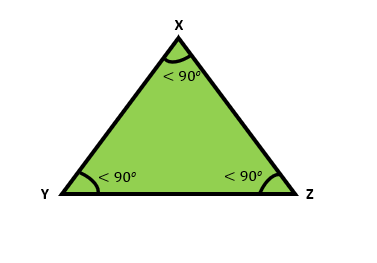2. Right Triangle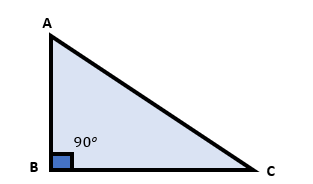3. Obtuse Triangle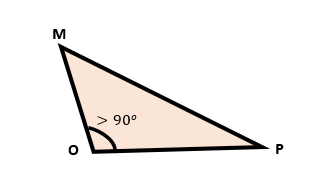## Classify Triangles Based on Lengths

Triangles can be classified based on the length of their sides.

1. Equilateral Triangles

• Equal-"lateral." Lateral means sides. Therefore, it means a triangle with equal sides (lengths).
• An equilateral triangle has three sides that are the same length. Therefore, they are congruent or of equal measure.
• An equilateral triangle is also an equiangular (equal angle measures) triangle because it has three sides of the same length.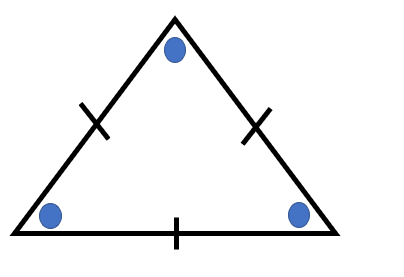2. Isosceles Triangles

• An isosceles triangle has two sides that are congruent or of the same length.
• An isosceles triangle has two congruent angles (base angles) because it has two sides of the same length.
• The other interior angle of an isosceles triangle is called the vertex angle.
• Isosceles Triangle Theorem states that if two sides of a triangle are congruent, the angles opposite those sides are congruent.
• The converse of the Isosceles Triangle Theorem states that if two interior angles of a triangle are congruent, the sides opposite those angles are congruent.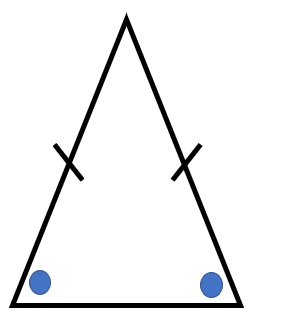3. Scalene Triangles

• A scalene triangle does not have any equal sides.
• A scalene triangle does not have any congruent angles because it does not have any equal sides.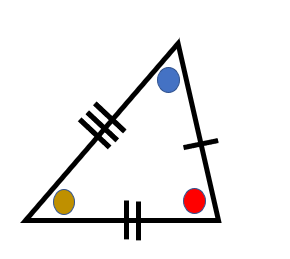## Sum of Interior Angles of a Triangle (Angle Sum Theorem)

The Angle Sum Theorem states that the sum of the three interior angles of any triangle is 180o.

Interior Angles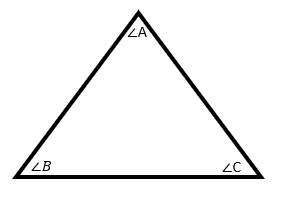Example:

Triangle DEF is a scalene triangle. What is the measure of $\angle D?$

## Exterior Angle Theorem

Any exterior angle of a triangle is equal to the sum of its two opposite (remote) interior angles.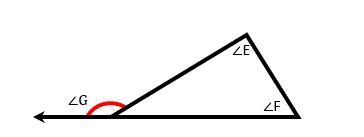Example:

Find the missing angle measure for the following triangle.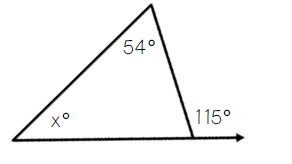## Triangle Inequality Theorem

• In a triangle, the sum of the lengths of any two sides is greater than the length of the third side. The difference in the lengths between any two sides is less than the length of the third side.
• If one side of a triangle is longer than the other side, then the angle opposite the longer side is larger than the angle opposite the other side.
• If one angle of a triangle is larger than the measure of the second angle, then the side opposite the larger angle is longer than the side opposite the second angle.
• Study the examples below:
1. Consider the given triangle $△ABC$. Give the range of values for the third side $\overline{)BC}$.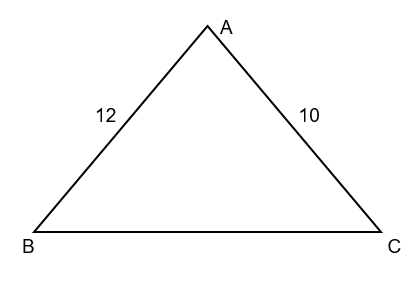$12-10

$2

Thus, the length of the third side $\overline{)BC}$ is any real number between $2$ and $22$.

2. Consider the given triangle $△XYZ$. Name the largest angle and the smallest angle.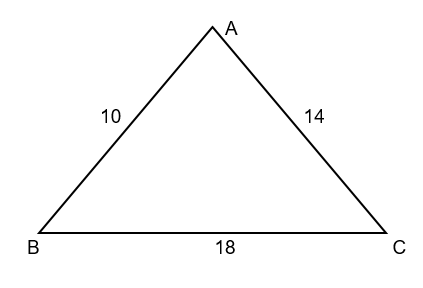The longest side $\overline{)BC}$ is opposite the largest angle which is $\angle A$.

The shortest side $\overline{)AB}$ is opposite the smallest angle which is $\angle C$.

## Solved Examples

Example 1. In a quadrilateral $ABCD$, the measures of  and $\angle D$ are in the ratio $1:2:3:4,$ respectively. Find the measures of the four angles.

Solution:

Let $x$ be the common factor.

$x+2x+3x+4x=360°$

$10x=360°$

$x=36$

Thus, the four angles are and $144°$.

Example 2. Find the measure of each angle of $△DEF$ if and $\angle F=\left(4x+10\right)°$.

Solution:

Use the Angle Sum Theorem.

$\angle D+\angle E+\angle F=180°$

$\left(3x\right)°+\left(5x-70\right)°+\left(4x+10\right)°=180°$

$12x-60=180$

$12x=240$

$x=20$

Thus, we have measures such as:

Example 3. The vertex angle of an isosceles triangle is $15°$ more than a base angle. Find the measure of each interior angle.

Solution:

Let $x$ be the measure of a base angle and $\left(x+15\right)°$ be the vertex angle.

$x+x+x+15=180$

$3x=165$

Each base angle is $55°$ and the vertex angle is $70°$.

## Cheat Sheet

• The sum of all interior angles of a convex polygon can be computed using the equation $S=\left(n-2\right)×180°$
• The measure of each interior angle of a regular polygon can be computed using the formula $\theta =\frac{\left(n-2\right)180°}{n}$.
• The measure of each exterior angle of a regular polygon can be calculated using the formula $\theta =\frac{360°}{n}$.
• To determine whether the given set of side lengths can create a triangle, we use the concept of the Triangle Inequality Theorem.
• The sum of the measures of all interior angles of a triangle is $180°$.
• The sum of the measures of all exterior angles of a quadrilateral is $360°$.
• In a convex polygon, the sum of all exterior angles is equal to $360°$.

## Blunder Areas

• The sum of the two remote interior angles and the exterior angle is not equal to $180°$.
• A triangle cannot be formed if the three segments fail to satisfy the conditions in Triangle Inequality Theorem.
• In solving problems without any figures, it is suggested to sketch a fairly accurate diagram to help visualize the shape and the unknown measure to be solved.
• In solving for the measure of angles and side lengths, the value must be any real number greater than $0.$
• Any triangle has no interior angle greater than $180°$.